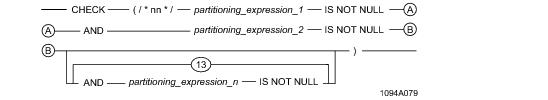# 15.00 - Partitioning CHECK Constraint for Multilevel Partitioning - Teradata Database

prodname
vrm_release
15.00
category
User Guide
featnum
B035-1094-015K

### Partitioning CHECK Constraint for Multilevel Partitioning

A multilevel partitioned table has the following partitioning CHECK constraint, which the system stores in DBC.TableConstraints. Call this partitioning constraint form 3.where:

 Syntax element … Specifies the … nn number of levels, or number of partitioning expressions, in the multilevel partitioning. nn can range between 02 and 62, inclusive. partitioning_expression_1 the first multilevel partitioning level. partitioning_expression_2 the second multilevel partitioning level. partitioning_expression_n the nth multilevel partitioning level. Note that if the multilevel partitioning has 3 levels, there are 3 NOT NULL partitioning expressions in the implied constraint, if the multilevel partitioning has 10 levels, there are 10 NOT NULL partitioning expressions in the implied constraint, and so on.

Single‑level partitioned tables have a different implied table‑level partitioning CHECK constraint (see “Single‑Level Partitioning” on page 362).

You can use the following query to retrieve a list of tables and join indexes that have PPIs and their partitioning constraint text.

`     SELECT DatabaseName, TableName (TITLE 'Table/Join Index Name'),             ConstraintText`
`     FROM DBC.IndexConstraintsV`
`     WHERE ConstraintType = 'Q' `
`     ORDER BY DatabaseName, TableName;`

You can use a query like the following to retrieve partitioning constraint information for each of the multilevel partitioned objects.

`     SELECT * `
`     FROM DBC.TableConstraints`
`     WHERE ConstraintType = 'Q' `
`     AND   SUBSTRING(TableCheck FROM 1 FOR 13) >= 'CHECK (/*02*/'`
`     AND   SUBSTRING(TableCheck FROM 1 FOR 13) <= 'CHECK (/*15*/';`

The TableCheck column of DBC.TableConstraints contains the unresolved condition text for a table-level constraint check or implicit table-level constraint such as a partitioning constraint. The ConstraintType code for such an implicit table-level constraint is Q for an object with a partitioned primary index, where in the case of a multilevel partitioning, each of the partitioning levels for the index appears once in the order defined for the table in the text contained in TableCheck. See Data Dictionary for details.

Rows that violate this implied index CHECK constraint, including those whose partitioning expression evaluates to null, are not allowed in the table.

Assume you create the following table:

`     CREATE TABLE orders (`
`       o_orderkey      INTEGER NOT NULL,`
`       o_custkey       INTEGER,`
`       o_orderstatus   CHARACTER(1) CASESPECIFIC,`
`       o_totalprice    DECIMAL(13,2) NOT NULL,`
`       o_orderdate     DATE FORMAT 'yyyy-mm-dd' NOT NULL,`
`       o_orderpriority CHARACTER(21),`
`       o_clerk         CHARACTER(16),`
`       o_shippriority  INTEGER,`
`       o_comment       VARCHAR(79))`
`     PRIMARY INDEX (o_orderkey)`
`     PARTITION BY (RANGE_N(o_custkey   BETWEEN 0`
`                                       AND 49999 `
`                                       EACH  100),`
`                   RANGE_N(o_orderdate BETWEEN DATE '2000-01-01' `
`                                       AND     DATE '2006-12-31'`
`                                       EACH INTERVAL '1' MONTH))`
`     UNIQUE INDEX (o_orderkey);`

The partitioning CHECK constraint SQL text that would be stored in DBC.TableConstraints for this multilevel partitioned primary index is as follows.

`     CHECK (/*02*/ RANGE_N(o_custkey   BETWEEN 0 `
`                                       AND 49999 `
`                                       EACH  100) `
`                   IS NOT NULL`
`               AND RANGE_N(o_orderdate BETWEEN DATE '2000-01-01' `
`                                       AND     DATE '2006-12-31' `
`                                       EACH INTERVAL '1' MONTH) `
`                   IS NOT NULL )`

The maximum size of this partitioning CHECK constraint is 16,000 characters.